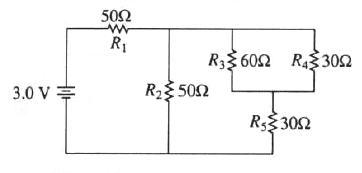GR 8677927796770177 | # Login | Register

GR9277 #32
Problem
 GREPhysics.NET Official Solution Alternate Solutions
\prob{32}In the circuit shown above, the resistance are given in ohms and the battery is assumed ideal with emf equal to 3.0 volts.

The resistor that dissipates the most power is

Electromagnetism}Circuits

Power is related to current and resistance by . The resistor that has the most current would be and (the equivalent resistance of all the resistors except for ), since all the other resistors share a current that is split from the main current running from the battery to . Since , the most power is thus dissipated through , as in choice (A).Alternate Solutions
 There are no Alternate Solutions for this problem. Be the first to post one!
NoPhysicist3
2017-03-23 10:13:45
Notice that the total resistance of the smallest circuit is 20 ohms. Therefore total resistance of the right vertical bar is 50 ohms, which is the same as R2. Since the current distributes between R2 end effective R (combined from R3, R4, R5) equally, we imply that the maximum current is at the R1, and therefore the maximum power is.tatitechno
2011-09-24 03:50:22
Hope we get one the these in the test !!
 scottsaw2013-08-24 11:05:35 Does anybody know if ETS ever re-uses old questions?
 mrshroom292013-09-19 22:25:32 Ive seen some similar questions between test. Then again I've seen test that are completely different from one another. If there is a possibility of being repeated or similar questions, they will be off the most recent tests that have been released.
 NoPhysicist32017-03-23 10:14:23 They stopped doing this after the cheating in china was revealed.Notice that the total resistance of the smallest circuit is 20 ohms. Therefore total resistance of the right vertical bar is 50 ohms, which is the same as R2. Since the current distributes between R2 end effective R (combined from R3, R4, R5) equally, we imply that the maximum current is at the R1, and therefore the maximum power is.LaTeX syntax supported through dollar sign wrappers $, ex.,$\alpha^2_0$produces . type this... to get...$\int_0^\infty\partial\Rightarrow\ddot{x},\dot{x}\sqrt{z}\langle my \rangle\left( abacadabra \right)_{me}\vec{E}\frac{a}{b}\$